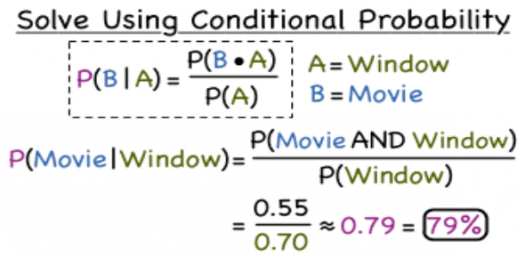# Conditional Probability Trivia Quiz

10 Questions | Total Attempts: 135SettingsThe concept of conditional probability is one of the most fundamental and one of the most important in probability theory. It is a measure of the probability of an event, with some specific situation occurring, given that another event has taken place.

• 1.
What can be used to reverse conditional probability?
• A.

Baye's theorem

• B.

Basic Probability

• C.

Reverse Probability

• D.

Omega theorem  of events

• 2.
The conditional probability of A given B is defined as the quotient of the probability of the joint of events A and B, and the probability of B. Whose definition is this?
• A.

Damien

• B.

Hopf

• C.

Kolmogrov

• D.

Bryan

• 3.
Which of these authors prefers to introduce conditional probability as an axiom of probability?
• A.

Conti

• B.

De finetti

• C.

Kolmogrov

• D.

Axiel Sappach

• 4.
What is a specified subset of an outcome called?
• A.

Space

• B.

Event

• C.

Total outcome

• D.

Expected outcome

• 5.
Which of these describes the behavior of an event?
• A.

Space

• B.

Laws of Indices

• C.

Law of large numbers

• D.

Law of possible outcome

• 6.
Probability is essential to what type of data analysis?
• A.

Qualitative

• B.

Graphical

• C.

Pictorial

• D.

Quantitative

• 7.
Which of these is not a central subject of probability?
• A.

Stochastic Process

• B.

Space and Event

• C.

Probability Distribution

• D.

Discrete random variable

• 8.
Which of these was a discovery in the twentieth century in Physics?
• A.

Quantum-Probability Relationship

• B.

Analytical work of space floating

• C.

Deterministic nature of theory

• D.

Probabilistic nature of physical phenomena

• 9.
Who published a book on Probability in 1657 during the early days of probability discovery?
• A.

Pierre Laplace

• B.

Blaise Pascal

• C.

Christiaan Huygens

• D.

Kolmogrov

• 10.
Who completed the research on mordern day "classic interpretation"?
• A.

Pierre Laplace

• B.

Kolmogrov

• C.

Pierre de Fermat

• D.

Blaise Pascal

Related TopicsBack to top